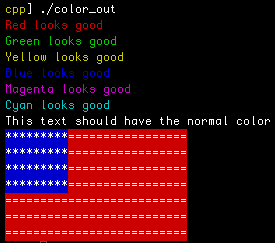```    1 #include <iostream>
2
3 using namespace std;
4
5 int main (int argc, char const *argv[]) {
6   const char COL_RESET[] = "\x1b[0m";
7
8   // Foreground colors are in form of 3x, bacground are 4x
9   const char RED[]     = "\x1b[31m";
10   const char GREEN[]   = "\x1b[32m";
11   const char YELLOW[]  = "\x1b[33m";
12   const char BLUE[]    = "\x1b[34m";
13   const char MAGENTA[] = "\x1b[35m";
14   const char CYAN[]    = "\x1b[36m";
15
16   cout << RED     << "Red looks good" << endl;
17   cout << GREEN   << "Green looks good" << endl;
18   cout << YELLOW  << "Yellow looks good" << endl;
19   cout << BLUE    << "Blue looks good" << endl;
20   cout << MAGENTA << "Magenta looks good" << endl;
21   cout << CYAN    << "Cyan looks good" << COL_RESET << endl;
22   cout << "This text should have the normal color" << endl;
23
24   // Cheap American flag, ASCII style.
25   cout << "\x1b[44m" << "*********"
26        << "\x1b[41m" << "=================" << endl;
27   cout << "\x1b[44m" << "*********"
28        << "\x1b[41m" << "=================" << endl;
29   cout << "\x1b[44m" << "*********"
30        << "\x1b[41m" << "=================" << endl;
31   cout << "\x1b[44m" << "*********"
32        << "\x1b[41m" << "=================" << endl;
33   cout << "==========================" << endl;
34   cout << "==========================" << endl;
35   cout << "==========================" << COL_RESET << endl;
36
37   return 0;
38 }```

## Output